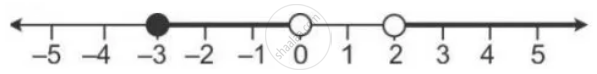Share

Books Shortlist

# Illustrate the Set {X: -3 ≤ X < 0 Or X > 2, X ∈ R} on the Real Number Line. - ICSE Class 10 - Mathematics

ConceptRepresentation of Solution on the Number Line

#### Question

Illustrate the set {x: -3 ≤ x < 0 or x > 2, x ∈ R} on the real number line.

#### Solution

Graph of solution set of -3 ≤ x < 0 or x > 2

= Graph of points which belong to -3 ≤ x < 0 or x > 2 or both

Thus, the required graph is:Is there an error in this question or solution?

#### APPEARS IN

Solution Illustrate the Set {X: -3 ≤ X < 0 Or X > 2, X ∈ R} on the Real Number Line. Concept: Representation of Solution on the Number Line.
S